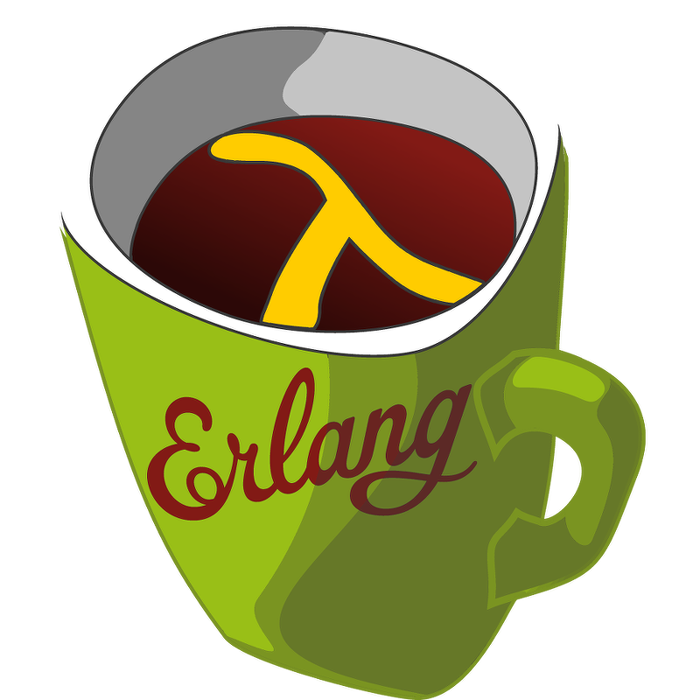This week's LFE Friday was translated with permission from the Erlang Thursday series by Steven Proctor. This week's translator: Robert Virding.

Today's LFE Friday is on digraph:in_neighbors/2.

`digraph:in_neighbors/2` takes a graph `G`, and a vertex `V`, and will return a list of all the vertices that have edges originating from them that are directed toward the vertex `V`.

We will continue working with the graph from last week's post on digraph:get_path/3.

``````> (set graph (digraph:new))
#(digraph 8207 12304 16401 true)
> (set v-1 (digraph:add_vertex graph 'v-1))
v-1
> (set v-2 (digraph:add_vertex graph 'v-2))
v-2
> (set v-3 (digraph:add_vertex graph 'v-3))
v-3
> (set v-4 (digraph:add_vertex graph 'v-4))
v-4
> (set e-1 (digraph:add_edge graph v-1 v-2))
(\$e . 0)
> (set e-2 (digraph:add_edge graph v-2 v-3))
(\$e . 1)
> (set e-3 (digraph:add_edge graph v-3 v-4))
(\$e . 2)
> (set e-4 (digraph:add_edge graph v-2 v-4))
(\$e . 3)
> (set e-5 (digraph:add_edge graph v-4 v-1))
(\$e . 4)``````

With that graph setup again, we can now find the `in_neighbors` of different vertices in our graph.

``````> (digraph:in_neighbours graph v-4)
(v-2 v-3)
> (digraph:in_neighbours graph v-1)
(v-4)
> (digraph:in_neighbours graph v-2)
(v-1)``````

So for vertex `v-4` we see the return value of `(v-2 v-3)` and for `v-1` we have an inbound neighbor of `v-4`, and for `v-2` we have the inbound neighbor of `v-1`.

## digraph:out_neighbors/2

The `digraph` module also contains the function digraph:out_neighbors/2, which returns a list of the vertices that a the given vertex "points to" with its edges in the directed graph.

``````> (digraph:out_neighbours graph v-2)
(v-4 v-3)
> (digraph:out_neighbours graph v-4)
(v-1)
> (digraph:out_neighbours graph v-1)
(v-2)``````

We can see from the picture of our graph that `v-2` has edges that "point to" the vertices `v-3` and `v-4`, and if we look at the result of `digraph:out_neighbors/2`, we get the result of the vertices `v-3` and `v-4`.

In this case we get the list of vertices where `v-4` is first and `v-3` is second, but that may not be the case, as the documentation states that the the edges are "in some unspecified order", which holds true of `digraph:in_neighbors/2` as well.

- Proctor, Robert

13 November 2015

tutorials